﻿ The Subwoofer DIY Page - Semi-Inductance
 The Subwoofer DIY Page Semi-Inductance 24 October 2018 Subwoofer alignments (a fancy word for the combination of box size, vent lengths, etc.) are typically designed using the basic T/S parameters of the driver that's being used. These parameters are Vas, Qts (which is a combination of Qes and Qms), Fs and Le.   The problem here is that Le, the driver's inductance (usually measured at 1kHz for subwoofer drivers) is assumed to remain constant, and it can actually vary quite a bit with frequerncy, and particularly so for the subwoofer drivers with long voice coils and little or no attempt in their design to reduce their inductance. As most box design programs assume that Le remains constant, the end result is that the measured performance of any subwoofers that were designed using these programs can vary signficantly from the predicted performance, if the driver's inductance varies significantly from its published value. The "semi-inductance" model is an attempt to address this issue.  A few more parameters are defined and used in this model to account for the driver's varying inductance, and as a result any subwoofers that are designed using this model will have a measured performance that's much more in line with what is predicted. The additional parameters that are used in the semi-inductance model are as follows:Re' - voice-coil / AC shorting resistance (Ohms)Leb -voice-coil inductance outside gap (mH)Le - voice-coil inductance inside gap (mH)Ke - voice-coil semi-inductance (sH)Rss - eddy-current losses (Ohms) These additional parameters are not usually published for subwoofer drivers, but they can be derived from their measured impedance curves. Example:Given below is the Hornresp input screen for a simple sealed box that's being designed for one of the Infinity 122.7W drivers I happen to have on hand. The box is being designed using the driver's measured T/S parameters, with includes a measured inductance of 3mH at 1kHz.   The box size is 56 liters, and it is defined to be 37 cm deep.  The parameters S1 and S2 are being used to define the box's baffle (the panel on which the driver is mounted).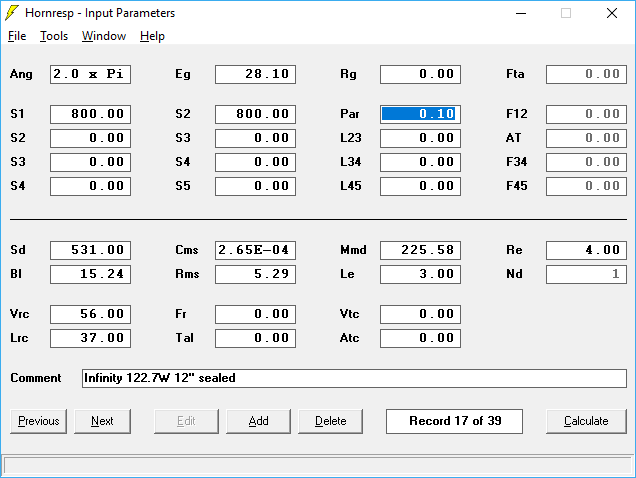So far, so good.  Let's see what the predicted performance of this sealed subwoofer looks like.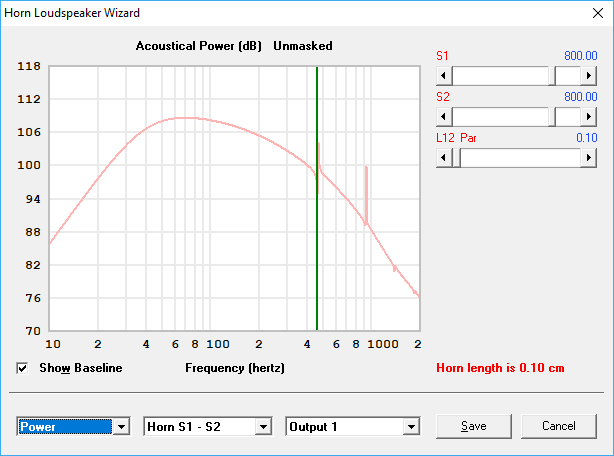Now, let's use the semi-inductance parameters to get an idea of what the actual performance of this subwoofer is going to be like.  To do this, I will go back to Hornresp's "Input Parameters" page, and double-click on the value for Le to bring up a separate dialog box to enter the semi-inductance parameters.  I had previously measured these to be as follows:Re'  = 4.507 OhmsLeb = 1.540 mHLe = 6.652 mHKe = 0.344 sHRss = 150.761 Ohms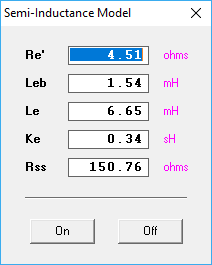Now, let's see what difference that made to the predicted performance of this particular subwoofer alignment...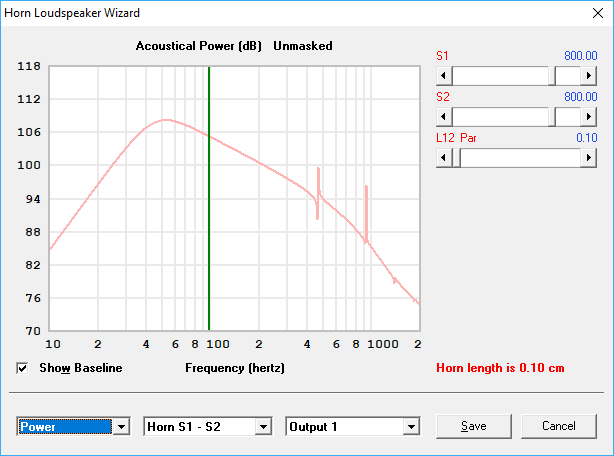Now that looks a bit different.  The smooth hump of the response predicted by the the use of the basic T/S parameters is now replaced by a sharper hump when the semi-inductance model is used. There's now an 8dB difference between the subwoofer's peak response and its response at 200 Hz, compared to ~3dB predicted by the basic model. Let's switch off the semi-inductance model for a moment to compare the two responses in one image: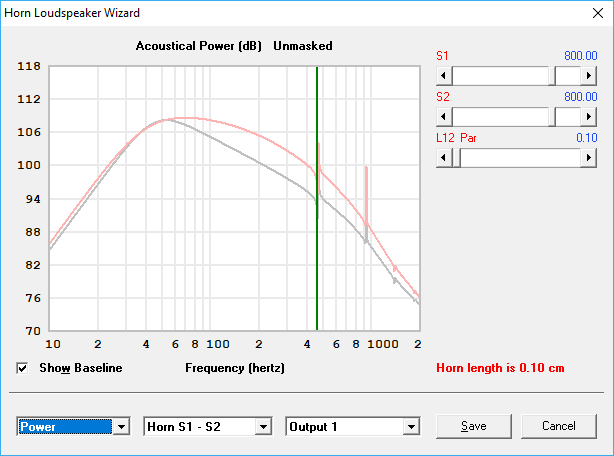In red is the response predicted by the basic T/S model - the response predicted by the semi-inductance model is in grey.  The two responses start to deviate from each other as low at 55 Hz.  There's also some minor deviation at very low frequencies, but that difference in performance between 55 Hz and 200 Hz is what is going to be most audible, and therefore what's going to make the most difference.  Note: this particular driver was designed for car audio duty, and the response predicted by the semi-inductance model suggests that it might be a good fit for that purpose.  The predicted frequency response does suggest though that a low-pass filter at a higher frequency than the usual 80 Hz can be used, particularly when you take a car's "cabin again" into effect. There will only be minor differences in predicted performance between the basic t/s model and the semi-inductance model if the driver has a short coil and/or very low inductance. However that small difference can impact some of the decisions taken during the design process.  Illustrated below for example is the predicted performance difference for my POC3 TH build when the semi-inductance model is used (grey).  If I had known about this back when I designed the POC3 TH, I would have opted to use a larger mouth, to address the drooping performance in the passband (40 Hz - 120 Hz)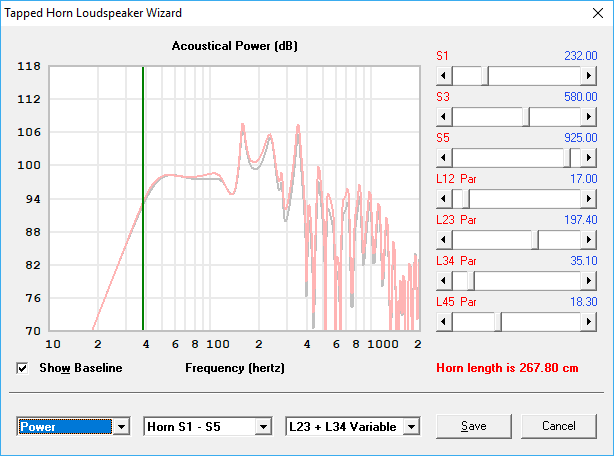Resources: "Electrodynamic Transducer Model Incorporating Semi-Inductance and Means for Shorting AC Magnetization"Knud Thorborg, Claus Futtrup Stephen Bolser's Semi-Inductance Calculator Spreadsheet Brian Steele 24 October 2018